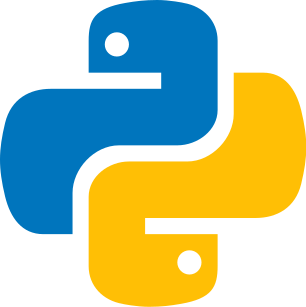Codistwa

# Practice Recursion / recursive functions - Quiz

algorithmfundamentals

## level 1

You've read the guide, now test your knowledge of Recursion / recursive functions! If you have any doubt, you can go back to the guide.### Question 1

What is the correct form of a factorial function?

1python
1def factorial(n):
2    if n == 1:
3        return n
4    else:
5        return n * factorial(n - 1)
6
7print(factorial(5))
2python
1def factorial(n):
2    return n * factorial(n - 1)
3
4print(factorial(5))
Select...

### Question 2

What is the time complexity of this recursion function?python
1def power(base, exponent):
2    if exponent == 0:
3        return 1
4    return base * power(base, exponent - 1)
5
6print(power(2, 3))
Select...

### Question 3

Which comic strip is recursive?

1
2
Select...